# Addition And Subtraction Of Vectors

Go back to  'Vectors and 3-D Geometry'

You will be able understand the discussion that follows very clearly only if you try to visualise everything physically. Everything about vectors will then automatically fall in place in your mind.

(A)  ADDITION OF VECTORS : TRIANGLE / PARALLELOGRAM LAW

Most of you will already be very familiar with how to add vectors, from your study of physics.

Consider two vectors $$\vec a\; and\; \vec b$$ which we wish to add. Let

$\vec c = \vec a + \vec b$

Thus,$$\vec c$$ should have the same effect as $$\vec a\; and\; \vec b$$ combined. To find the combined effect of $$\vec a\; and\; \vec b$$, we place the initial point of $$\vec b$$ on the end-point of $$\vec a$$(or vice-versa):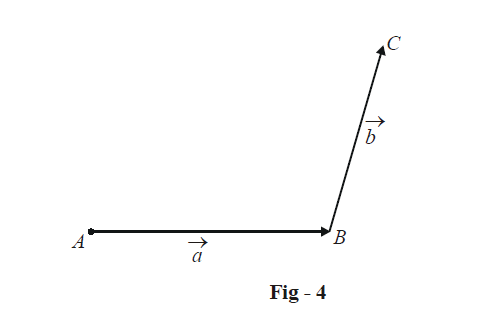A person who starts at point A and walks first along $$\vec a$$ and then along $$\vec b$$ will reach the point C. Thus, the combined effect of $$\vec a\; and\; \vec b$$ is to take the person from A to C, i.e,$$\vec a + \vec b = \vec c$$ should be the vector $$\overrightarrow {AC}$$ :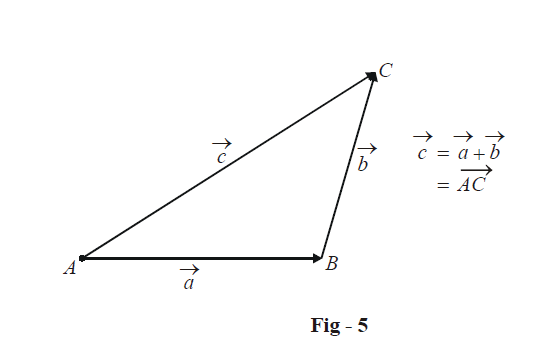In general, we see that to add two vectors, say $$\vec a\,{\rm{and}}\,\vec b$$ , we place the initial point of one of them, say $$\vec b,$$at the end-point of the other, i.e.,$$\vec a$$ . The vector $$\vec a + \vec b$$ is then the vector joining the tip of to $$\vec a$$ the end-point of $$\vec b$$ . This is the triangle law of vector addition. $$\vec a\,{\rm{and}}\,\vec b$$ can equivalently be added using the parallelogram law; we make the two vectors co-initial and complete the parallelogram with these two vectors as its sides: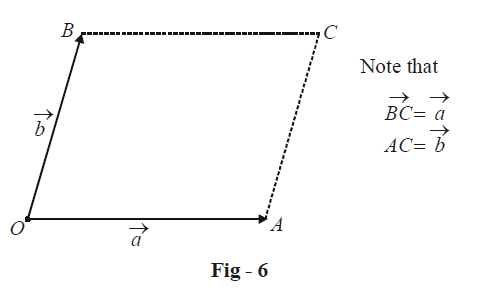The vector  $$\overrightarrow {OC}$$ then gives us the sum of $$\vec a\,{\rm{and}}\,\vec b$$.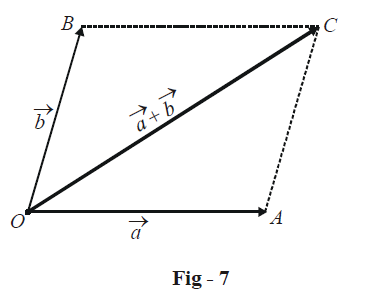Note that the triangle and the parallelogram law are entirely equivalent; they are two slightly different forms of the same fundamental principle.

(a) Existence of identity:            For any vector $$\vec a$$,

$\vec a + \vec 0 = \vec a$

so that $$\vec 0$$ vector is the additive identity.

(b) Existence of inverse:              For any vector  $$\vec a$$,

$\vec a + \left( { - \vec a} \right) = \vec 0$

and thus an additive inverse exists for every vector.

(c) Commutativity:                       Addition is commutative; for any two arbitrary vectors $$\vec a\,\,{\rm{and}}\,\,\vec b$$  ,

$\vec a\, + \vec b\, = \vec b + \vec a$

(d) Associativity:                           Addition is associative; for any three arbitrary vectors $$\vec a,\vec b\,\,{\rm{and}}\,\,\vec c$$ ,

$\vec a + \left( {\vec b + \vec c\,} \right) = \left( {\vec a + \vec b} \right) + \,\,\vec c$

i.e, the order of addition does not matter.

Verify this explicitly by drawing a vector diagram and using the triangle / parallelogram law of addition.

(B) SUBTRACTION OF VECTORS : An extension of addition

Consider two vectors $$\vec a\,\,{\rm{and}}\,\,\vec b$$ ; we wish to find $$\vec c$$ such that

$\vec c = \vec a - \vec b$

We can slightly modify this relation and write it as

$\vec c = \vec a + \left( { - \vec b} \right)$

and thus subtraction can be treated as addition. To do this, we first reverse the vector $$\vec b$$ to obtain $$- \,\vec b$$ and then use the triangle / parallelogram law of addition to add the vector $$\vec a$$ and (–$$\vec b$$):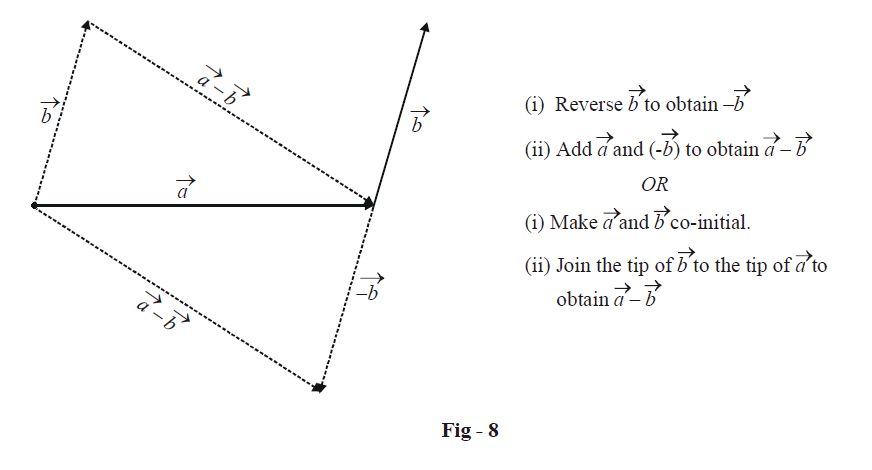Joining the tip $$\vec b$$ of to the tip of $$\vec a$$(if $$\vec a$$ and $$\vec b$$are co-initial) also gives us $$\vec a - \vec b.$$

Note that from the triangle law, it follows that for three vectors  $$\vec a,\vec b\,\,{\rm{and}}\,\,\vec c$$ representing the sides of a triangle as shown,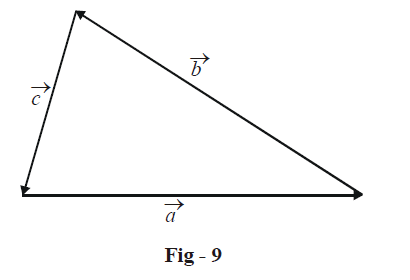we must have

$\vec a + \vec b + \vec c = \vec 0$

In fact, for the vectors $${\vec a_i},\,\,i = 1,2.....n,$$ representing the sides of an n-sided polygon as shown,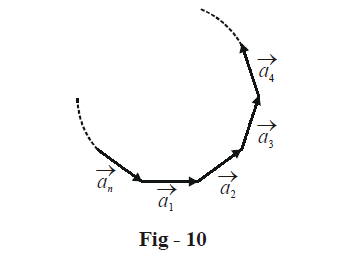we must have

${\vec a_1} + {\vec a_2} + ....... + {\vec a_n} = \vec 0$

since the net effect of all vectors is to bring us back from where we started, and thus our net displacement is the zero vector.

Vectors
grade 11 | Questions Set 1
Vectors